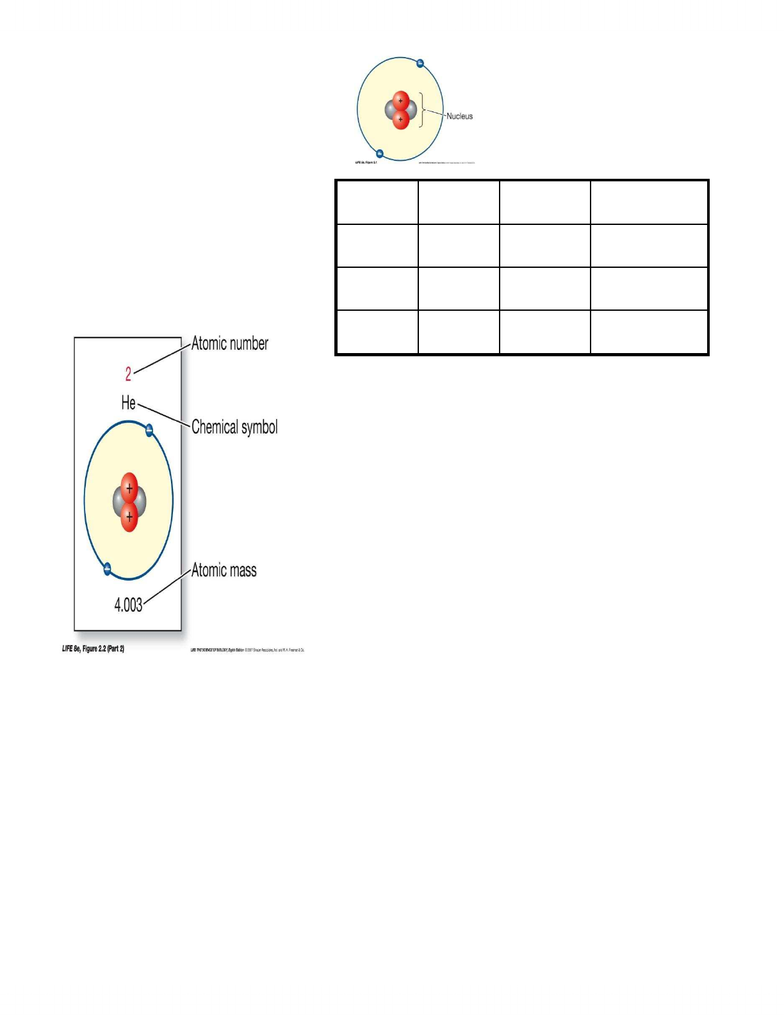Textbook Notes (280,000)
CA (170,000)
UTSC (20,000)
BIOA01H3 (200)
Chapter 2

# BIOA01H3 Chapter Notes - Chapter 2: Atomic Mass, Atomic Number, Covalent Bond

Department
Biological Sciences
Course Code
BIOA01H3
Professor
Mark Fitzpatrick
Chapter
2

This preview shows page 1. to view the full 4 pages of the document.BIOA01 t LEC3 CHAPTER 2
x All matter is composed of atoms
x mass of one electron - 9 10t28
x Protons + Neutrons = Atomic Mass
x Protons + Electrons = Charge
x Elements t pure substance
containing only on kind of atom
x Elements are arranged in the
periodic table
x Six main elements t Hydrogen,
Carbon, Nitrogen, Oxygen, and
Phosphorus
x The number of protons identifies an element
x Number of protons = atomic number
x All elements except hydrogen have one or more neutrons
x Mass number is the number of protons + number of neutrons
x Mass number = mass of atom if Daltons
x Isotopes t forms of an element with different numbers of
neutrons, thus different mass numbers
x Atomic weight: average of mass numbers of isotopes in their
normally occurring proportions
x Radioisotopes are unstable; they give off energy in the form of
alpha, beta, and gamma radiation from the nucleus.
This radioactive decay transforms the atom, including
changes in the number of protons
Energy from radioactive decay can interact with
surrounding material
Radioisotopes can be incorporated into molecules and
^P_}oo
x Reactivity of atoms = electrons
o The number of electrons determines how atoms will interact
o Chemical reactions involve changes in the distribution of electrons between atoms
x Locations of electrons in an atom are described by orbitals.
o Orbital t region where electron is found at least 90 percent of the time.
o Orbitals occur in series called electron shells or energy levels.
o Orbitals are filled in a specific sequence.
x Reactive atoms have unpaired electrons in their outermost (valence) shell.
x Atoms can share electrons, loose or gain electrons, resulting in atoms bonded together to form
molecules.
Type
Charge
Mass (da)
Info Provided
Proton
+1
1
Atomic number
Neutron
0
1
Isotope
Electron
-1
negligible
Reactivity
www.notesolution.com